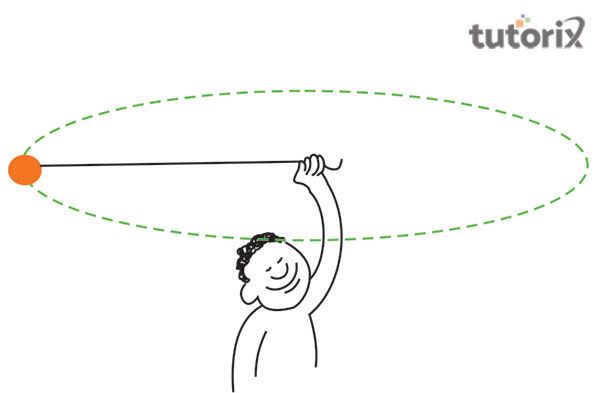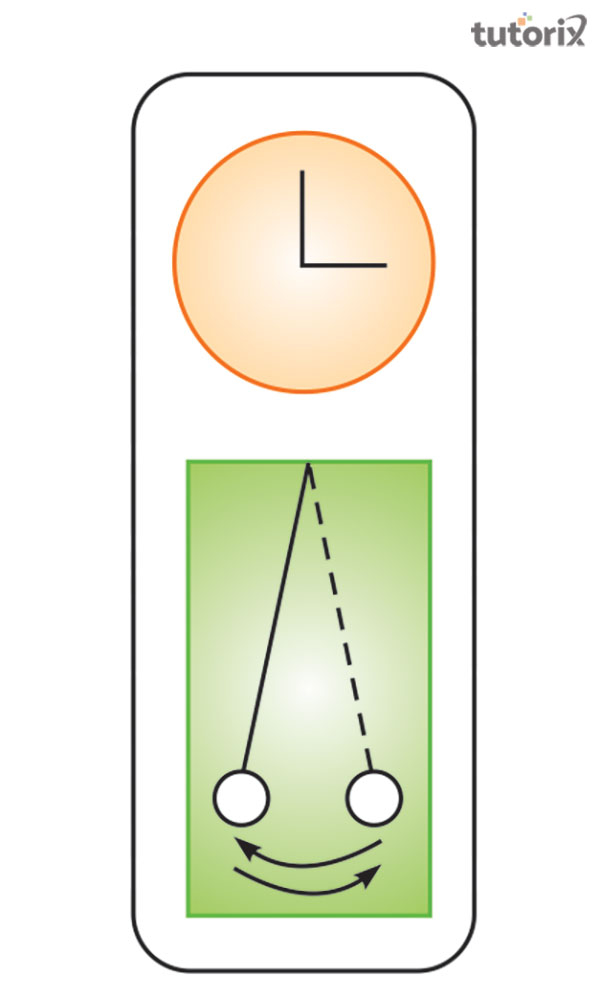# Types of Motion Measurement

## Introduction

The multitudes of motions that are found in nature are associated with the simple functionality of change eventuating in the position of a certain product or object. It so happens that as the object continues to alter its initial position with respect to time the object is said to be in motion otherwise the object is concluded to be in a state of rest. In context to the case of motion, the aspect f measurement differs from one type of motion to the other. In terms of measuring the state of the motion the objects in consideration are quantified to gather desired results.

## What is defined as motion?

Every object that is visible around in nature is capable of attaining a certain state of motion. According to Latorrraca et al. (2017), the motion of the body can be determined through the change in position that is visible with respect to time. For example, If It is observed that an insect is changing its position from one point to another it can be concluded that the insect is in a state of motion.

However, there are cases where the motion is not visible through the naked eye. For example, the earth is rotating and revolving at a very high speed however it can be perceived or seen through the naked eye. Therefore, it can be inferred that the state of motion is also established with respect to the distinct initial and final position of the object.

## Kinds of motion

The motion of an object displays the alteration in position. However, the motion is classified into multiple sections.

• Rectilinear motion: In context to rectilinear motion, it can be established that the objects are noticed to be moving along a course of a single path. One of the motion common examples of this motion is observed in the marching or parade of the soldiers, movement of cars in a single lane or animals of the same group moving in a herd.

• Circular motion: Certain objects are noted to be in a state of motion however these objects are fixed in a distinct location. A person swinging a ball also experiences a uniform circular motion (physics, 2022). This motion is typically noticed in the motion of a fan. In such a case, the fan is fixed at one point however it adheres to a circular motion.Figure 1: Circular motion

In context to the circular motion, it is observed that the bodies or objects course through a rotational motion that does not require them to alter their position from one point to another . There are various examples of this motion to be found in the surroundings. For example, a ferry wheel, satellites also display this motion.

Periodic motion: This type of motion is most commonly observed, in case of a pendulum. Although it is stuck at one position it still tens to dwindle from its fixed position. Therefore it is understood that in this particular motion the movement of the object is repeated over a course of time .Figure 2: Oscillatory motion

This specific type of movement is also termed oscillation because the motion repeats itself after a distinct episode of time. The most common example of periodic motion is sound in the pendulum locks and the table fans. An individual on a swing also experiences a periodic motion.

## Measurement systems

Measurements are being conducted over a long period. Since ancient times different aspects are measured for certain results that including the aspects of motion. The aspect of motion and other aspects are measured as per the conventional system of measurements, that is, the measurements are conducted as per the conventions of the people. This however is a very significant demerit of this aspect of measurement because such kind of measurement varies from one person to another. These conventional systems include a hand span, length of an arm, and footsteps and cubit.

Therefore, a standard unit of measurement is observed to gather accurate results in the context of motion. The efficiency of this type of measurement resides in the fact that this measurement does not vary from one person to another and is standardized overall. These units are also termed International System of Units or simple, S. I. units. The distance coursed by an object is calculated with the SI unit, meter (m).

There are however certain points that are to be considered while measuring with accuracy. For example, a proper device must be taken for the measurement of distinct objects and the measuring devices are not to be generalized for every measurement. For example, curvy lines will be able to be measured accurately with the help of a straight scale. In the case of measurement, it is crucial to measure that the initial position is aligned with zero or the absolute initial notation. The observers are also needed to align their eyes properly for the obtainment of accurate results.

## Conclusion

It can be safely concluded that almost all the objects that are found in nature are one way or another in a constant state of motion whether the observable movement is noticed with respect to time’ or from its change in position. The relative changes in motion are calculated either by the conventional ways that vary from one person to another or by standardized units. These aspects of measurements generally consist of two things one is the unit and the number of the units.

## FAQs

Q1. What are the types of motion?

Ans. The motion is classified into three types in general. The rectilinear motion, circular motion and the periodic motion govern various types of motion.

Q2.What are the examples of oscillatory motion?

Ans. Oscillatory motion is observed in the table fan. A person swinging on a swing also experiences this kind of motion.

Q3.What are the two kinds of linear motion?

Ans. Linear motion can further be subdivided into two types. Rectilinear motion is where objects take a straight path and curvilinear motion is where the objects follow a curved path.

Q4. What are the parameters of measurement of motion?

Ans. Motion is measured under four distinct parameters. Distance, speed, displacement and time are those parameters of measurement.